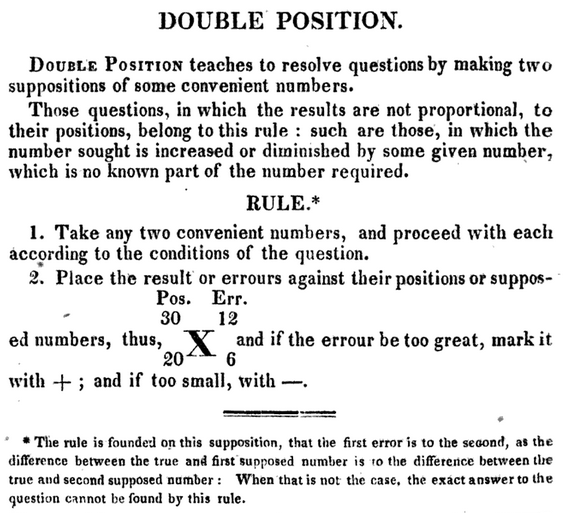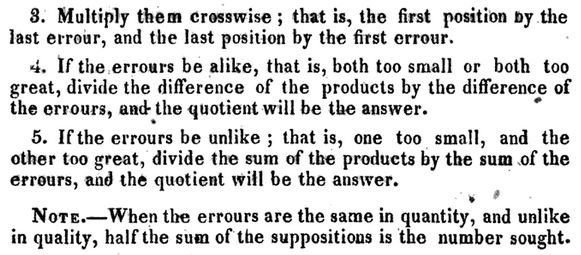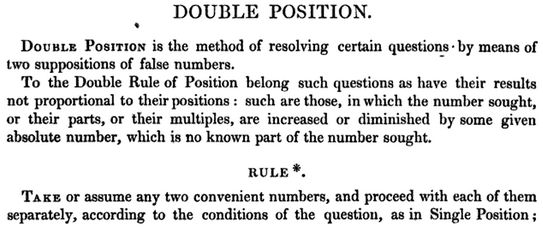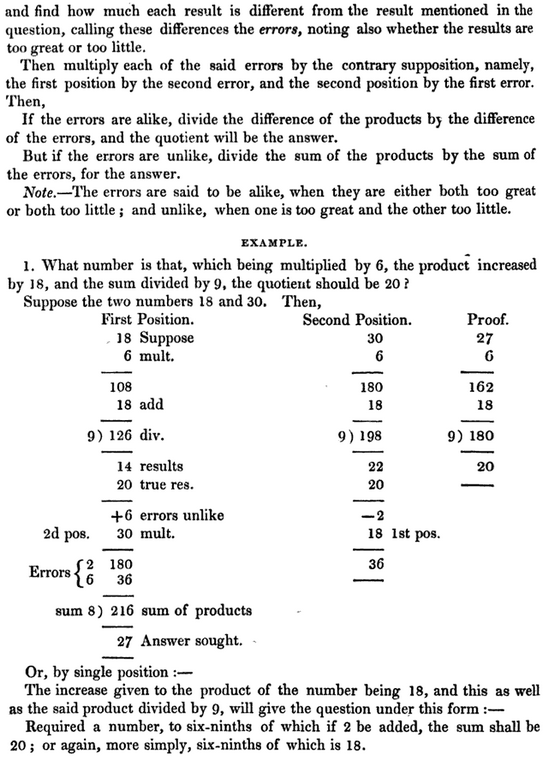# The Method of False Position: Old and New

#### (An archive question of the week)

Last time, as part of our series on estimation, we looked at some numerical methods for solving equations approximately. I mentioned the Method of False Position, but when I looked for more detailed expositions in our archive, I realized that in a sense it is really two different things, and we primarily cover the form that is not a method of approximation. Today I will focus on two answers from the archive, one related to each aspect of the method, in order to dig more deeply into both.

## Simple False Position (Regula falsi)

This method goes back to the ancient Egyptians or Babylonians; the name comes from the fact that we start by supposing (positing) a solution (though we know it is false), and using the information obtained to get a new answer — which, for some (linear) problems, will in fact be the exact answer, but for others (non-linear) will just be a better approximation. In the latter form, it is similar to the Bisection Method I discussed last time, but rather than getting a mere “up or down” indication from the function, we have more information, so we can move faster.

The ancient form of the method (for linear problems) came up in this question from 2004:

The Method of False Position

There is a quantity such that 2/3 of it, 1/2 of it, and 1/7 of it added together becomes 33.  What is the quantity?  Solve the problem by the method of false position.

I know that using the method of false position, we are looking for the root of an equation, and need a way of making a guess that is better than our previous guess.

This is a classic problem of the Egyptian type, though Lynne didn’t mention such a source; it may be a direct translation from an Egyptian original, or a problem from the 19th century (see below), which explains its awkward wording. If you think about it, you will realize that it is really a simple algebra problem, from our perspective. The hard part is that it is written in terms of a sum of fractions; this is the only way the Egyptians could write fractions, as their notation only allowed for “numerators” of 1, apart from a special case for 2/3 or 3/4. Today, we would just combine the fractions and multiply by the reciprocal. There is no need for a numerical approximation, and in fact this method quickly gives the exact answer for such a problem.

Let's let 'x' be the unknown quantity, and we can write the following equation:

x*(2/3) + x*(1/2) + x*(1/7) = 33

Assume x = 42.  This is a convenient first guess because it is divisible by all of the denominators {3,2,7}, which makes our life a bit easier on the first step.

42*(2/3) + 42*(1/2) + 42*(1/7) = 28 + 21 + 6 = 55.

So our first guess of 42 is approximately too big by a factor of 55/33 = 5/3.  Our next guess is therefore

42*(3/5) = 126/5 or 25.2.

We plug this, our second guess, in for x in the equation above to obtain

(126/5)*(2/3) + (126/5)(1/2) + (126/5)(1/7)
= 84/5 + 63/5 + 18/5
= 165/5 = 33

which is exactly what we want it to be, and we've therefore found the value of x for which the equation is true.  There is of course a more direct method to solve the original equation using algebra techniques, but I think that this problem shows you how the method of false position works so that you can also apply it to cases where you cannot simply solve for x.

Both Lynne and Doctor Douglas appear to know False Position primarily as an approximation method, despite this being a classic problem for the linear form of the method. I added a reference to the following page, which I had written the previous year, about its (presumed) Egyptian origin and significance:

The Egyptians' Method of False Position

What we have used here is called “Simple False Position”, and applies specifically to problems of the form $$ax = b$$ (direct proportion). In it, we make one guess, and adjust the guess proportionally to the error in the result. As I see it, what was done above could just as well be described as multiplying by a common denominator to simplify the equation, and then dividing by the remaining coefficient. If so, then it is not all that different from modern methods. Their main difficulty was the lack of notation, which forced them to say everything in words.

The more general method, used to solve equations of the form $$ax + b = c$$, is called “Double False Position”, and is the precursor of the full approximation method we’ll look at below. In it, we make two guesses, and use a complicated formula to find the new “guess” lying between them, which for a linear equation will be the final answer. I’ll discuss this formula after we see it in an example of approximation.

## Approximation by Linear Interpolation

The best discussion I find of this is in the following question from 2006, where Jacob essentially discovered the core of the method himself:

A Way to Estimate the Square Root of Any Number

Today in math class, we learned about finding the square root of certain numbers, such as the square root of 36 = 6.  I found a way to find the approximate square root of any number, and I need to know why it works.

Example: the square root of 63.  To get the square root of 63 first you take the nearest two perfect squares, 49 and 64.  Take the square roots of those numbers, which are 7 and 8.  Set it up like this:

49   63   64
7    ?    8

Next we need to make a fraction.  Subtract 49 from 64 to get your denominator, 15.  To get your numerator you subtract the first two numbers, 63 - 49 = 14.  That leaves you with 14 over 15 or .93.  Since the square root of 63 is higher than 7 but less than 8 your whole number is 7.  Add .93 to 7 and get 7.93, close to the real square root of 63.

In my math class we always question why.  So, why does this work?

I love his original title for the question: “Wow! I have found it, now why does it work?” I think he is in a wonderful class.

I explained what he had done in terms of the graph:

What you've discovered is called "linear interpolation". It amounts to approximating a point on a graph by using a straight line that is close to the graph.

Draw the graph of the square root, y = sqrt(x). It passes through the points (49,7) and (64,8), since the square roots of 49 and 64 are 7 and 8 respectively. Between them, the graph is slightly curved.

If you draw a line between those two points, you'll find that the line is quite close to the graph of the square root. Here's a close-up of the line:

8 -------------------- o
/  |
o     |
/  |     |1
/     |h    |
/        |     |
7 -- o-----------+-----+
|           |     |
49          63    64
\_________/
63-49=14
\_______________/
64-49=15

Since 63 is 14/15 of the way from 49 to 64, h is 14/15 of the way from 7 to 8. That means the value of y, when x is 63, is 7 14/15. That's your estimate of the square root.

This method is often used for quick estimates; back before calculators, we were taught to use it to find values from trigonometric tables when we needed extra accuracy.

I was not satisfied with the rough “graph” I’d made, so I sent a better one:

Here's a picture of the full graph that I talked about in case you'd like to see it, with the triangle I drew in red.  You can see that the straight line is very close to the actual square root graph:Did you discover this on your own, or did you have some hints that led you in this direction? It's a very nice thing to have found!

Jacob replied,

I discovered it on my own and my teacher was amazed at the discovery. She had never heard of figuring it out in that way.  Thanks for your reply.  That makes sense.

Now, as I said, what he did was a common way to make an estimate from a table (it’s still used in countries where tables are used for trigonometry or logarithms); Jacob just did it once, so it is not yet an iterative method. Let’s look a little deeper to see how this could be turned into (a) a formula for Double False Position, and then (b) a method for iterative approximation.

## Double False Position: the formula

First, what is the formula? In looking for the square root of 63, Jacob first made two “false guesses”, 7 and 8, knowing that $$7^2 < 63 < 8^2$$, and then calculated that 63 is 14/15 of the way from 49 to 64, so that (if the curve were a straight line), x should be 14/15 of the way from 7 to 8. So, in general, to find the square root of n, we make two guesses a and b, and estimate that x should be $$\displaystyle a + \frac{n – a^2}{b^2 – a^2}\cdot (b – a)$$.

More generally, suppose we were searching for a zero of the function $$f(x)$$, and we find two numbers $$x_1$$ and $$x_2$$ that bracket the zero we want. Let’s call $$y_1 = f(x_1)$$ and $$y_2 = f(x_2)$$. Then the x-intercept is $$0 – y_1$$ out of $$y_2 – y_1$$ of the way from the first point to the second vertically, so our approximation will be $$\displaystyle \frac{-y_1}{y_2 – y_1}$$ of the way from $$x_1$$ to $$x_2$$. Our approximation is therefore $$\displaystyle x_1 + \frac{-y_1}{y_2 – y_1}\cdot (x_2 – x_1) = \frac{x_1 y_2 – x_1 y_1 – y_1 x_2 + y_1 x_1}{y_2 – y_1} = \frac{x_1 y_2 – y_1 x_2}{y_2 – y_1}$$. This is our formula. If the goal is not zero, the y‘s are taken to be errors (difference from the goal).

Ancient people (starting at least with the Chinese, Indian, and Islamic worlds, and, in fact, all the way into the 19th century!)  used this as a rote method for solving linear problems by using two guesses; this was called Double False Position, or just Double Position. (The word “position”, here, means something like “supposition”: we are twice “positing” a possible but false solution.) In my cursory examination of old books discussing this, I see some literally cross-multiplying (arranging the guesses x and the errors y at the ends of an X), some using something like our unsimplified formula instead. Some modified the rule when the two guesses were on the same side of the solution, rather than use signed numbers. Here is one example from 1827:

Pike’s System of Arithmetick Abridged: Designed to Facilitate the Study of the Science of Numbers, Comprehending the Most Perspicuous and Accurate Rules, Illustrated by Useful Examples : to which are Added Appropriate Questions, for the Examination of Scholars, and a Short System of Book-keepingHere’s another, from 1836:

A course of mathematics, Charles HuttonIt seems incredible today that such a cumbersome method should have been commonly taught; but algebra was considered too advanced for most students. This is just arithmetic!

## False Position as an iterative approximation method

Again, these versions of the method apply specifically to linear problems, and will give an exact answer that can be checked. But if we apply it repeatedly to a non-linear problem, we will get a better approximation at each step. Let’s take Jacob’s example and do that.

His work, again, used the formula

$$\displaystyle a + \frac{n – a^2}{b^2 – a^2}\cdot (b – a)$$;

applied to his example with n = 63, a = 7, b = 8, we had

$$\displaystyle 7 + \frac{63 – 7^2}{8^2 – 7^2}\cdot (8 – 7) = 7 + \frac{14}{15} = 7.9333$$.

Checking, we find that $$7.9333^2 = 62.9372$$, which is a little low, so the solution lies between 7.9333 and 8. Repeating using these two guesses, we have

$$\displaystyle 7.9333 + \frac{63 – 7.9333^2}{8^2 – 7.9333^2}\cdot (8 – 7.9333) = 7.9333 + \frac{63 – 62.9372}{64 – 62.9372}\cdot (8 – 7.9333)$$ $$\displaystyle = 7.9333 + \frac{0.0628}{1.0628}\cdot (0.0628) = 7.9333 + .0037 = 7.9370$$

as our new approximation; checking, we see that $$7.9370^2 = 62.996$$. That is quite close to 63. (A calculator gives the value 7.9372539… .)

More generally, we would repeat our formula $$\displaystyle x_{n+1} = \frac{x_{n-1} y_{n} – y_{n-1} x_{n}}{y_{n} – y_{n-1}}$$, or any equivalent form, and then pair this with the last guess for which y had the opposite sign.

This is, in essence, the method of False Position as used today as an approximation method. There are a number of variations on it.

Next week, I will discuss finding square roots by other methods that are more useful by hand.

This site uses Akismet to reduce spam. Learn how your comment data is processed.